Data Science Desktop Survival Guide by Graham WilliamsDesktop Survival Project Home Preface Data Science Introducing R R Constructs R Tasks R Strings R Read, Write, and Create Data Template Data Exploration Data Wrangling Data Visualisation Statistics ML Template ML Scenarios ML Activities ML Applications ML Algorithms Cluster Analysis Decision Trees Computer Vision Graph Data Privacy Literate Data Science Coding with Style Resources Bibliography Index

## Interpret RPart Decision Tree

 ```## n= 256 ## ## node), split, n, loss, yval, (yprob) ## * denotes terminal node ## ## 1) root 256 42 No (0.83593750 0.16406250) ## 2) Cloud3pm< 6.5 180 11 No (0.93888889 0.06111111) * ## 3) Cloud3pm>=6.5 76 31 No (0.59210526 0.40789474) ## 6) Pressure3pm>=1016 34 5 No (0.85294118 0.14705882) * ## 7) Pressure3pm< 1016 42 16 Yes (0.38095238 0.61904762) ## 14) WindDir3pm=ESE,SSE,W,WNW,WSW 17 5 No (0.70588235 0.29411765) * ## 15) WindDir3pm=ENE,N,NE,NNE,NNW,NW,S 25 4 Yes (0.16000000 0.84000... ```

The textual version of a classification decision tree is reported by rpart.

The legend, which begins with node) indicates that each node is identified by a number, followed by a split (which will usually be in the form of a test on the value of a variable), the number of observations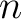at that node, the number of observations that are incorrectly classified (the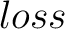), the default classification for the node (the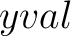), and then the distribution of classes in that node (the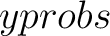) across No and Yes. The next line indicates that a “*” denotes a terminal node of the tree (i.e., a leaf node—the tree is not split any further at that node).

The actual tree starts with the root node labelled 1). observations and a default decision of No. There are 42 observations with Yes as the decision, so these are “lost” if we make the decision No for all observations. The probability of No is reported as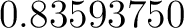(which is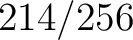) and of Yes is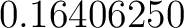(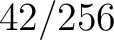).

The root node is split into two branches, nodes number 2 and 3. For node number 2, the split corresponds to those observations for which `Cloud3pm` is less than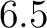. This accounts for 180 observations and whilst 11 of them are Yes the majority (with a proportion of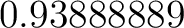) are No. We can read the remainder of the tree similarly. Node 3 is split into two other nodes, the second of which is split further until the terminal nodes.